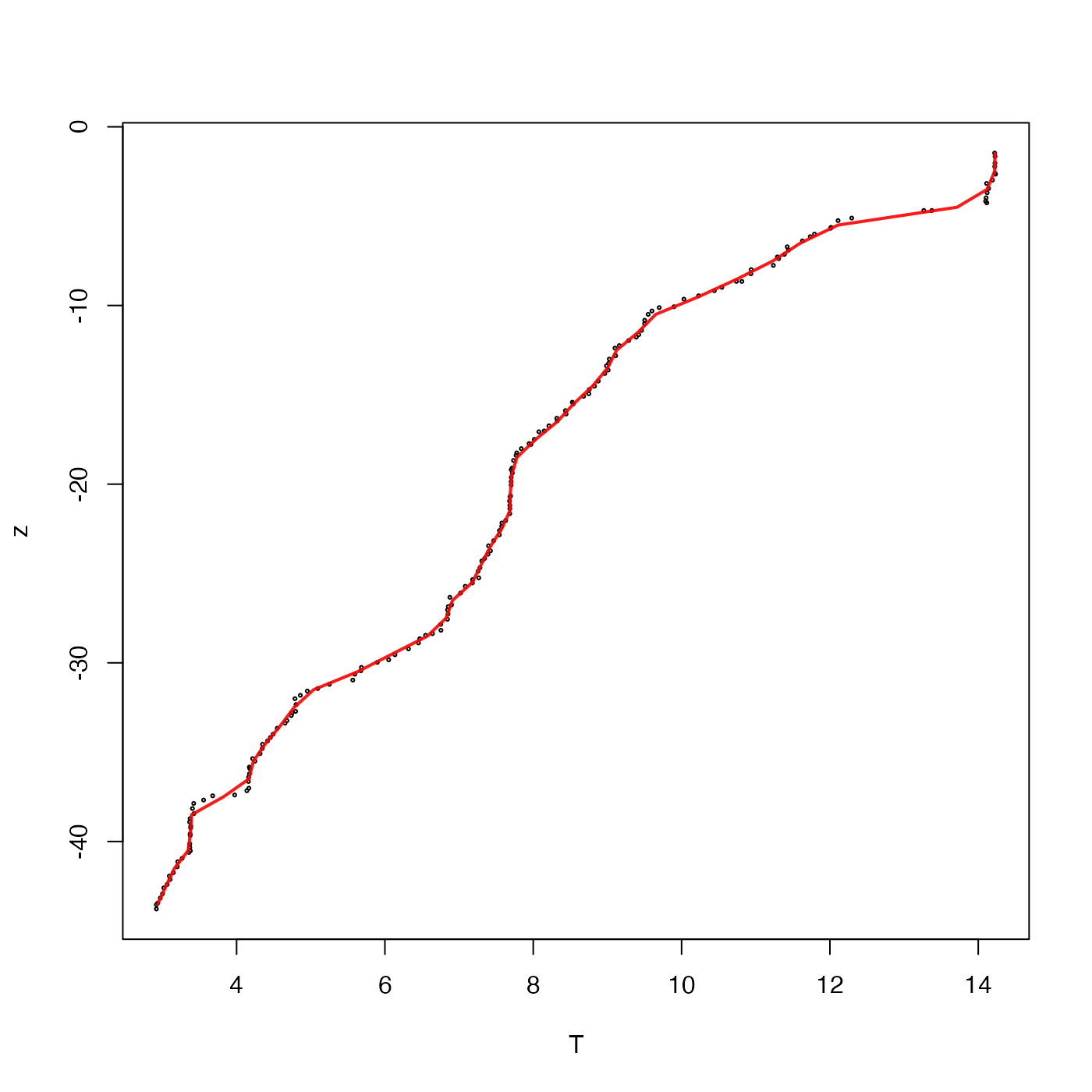Average the values of a vector f in bins defined on another vector x. A common example might be averaging CTD profile data into pressure bins (see “Examples”).

binMean1D(x, f, xbreaks)

## Arguments

x vector of numerical values. vector of numerical values. Vector of values of x at the boundaries between bins, calculated using pretty() if not supplied.

## Value

A list with the following elements: the breaks (xbreaks, midpoints (xmids) between those breaks, the count (number) of x values between successive breaks, and the resultant average (result) of f, classified by the x breaks.

Other bin-related functions: binApply1D(), binApply2D(), binAverage(), binCount1D(), binCount2D(), binMean2D()
library(oce)
lines(TT$result, TT$xmids, col='red')